## Chapter 14Fractional Numbers

So far in this book we have used only integers for numerical values. In this chapter you will see two methods for storing fractional values — ﬁxed point and ﬂoating point. Storing numbers in ﬁxed point format requires that the programmer keep track of the location of the binary point1 within the bits allocated for storage. In the ﬂoating point format, the number is essentially written in scientiﬁc format and both the signiﬁcand2 and exponent are stored.

### 14.1 Fractions in Binary

Before discussing the storage formats, we need to think about how fractional values are stored in binary. The concept is quite simple. We can extend Equation 2.6:(14.1)

For example,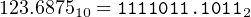because

 d−1 × 2−1 = 1× 0.5 d−2 × 2−2 = 0× 0.25 d−3 × 2−3 = 1× 0.125 d−4 × 2−4 = 1× 0.0625

and thus

 0.10112 = 0.510 + 0.12510 + 0.062510 = 0.687510 (14.2)

See Exercise 14-1 for an algorithm to convert decimal fractions to binary. We assume that you can convert the integral part and that Equation 14.1 is suﬃcient for converting from binary to decimal.

Although any integer can be represented as a sum of powers of two, an exact representation of fractional values in binary is limited to sums of inverse powers of two. For example, consider an 8-bit representation of the fractional value 0.9. From

 0.111001 = 0.8984375010 0.111001 = 0.9023437510

we can see that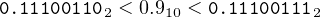(14.3)

In fact,(14.4)

where 1100 means that this bit pattern repeats forever.

Rounding oﬀ fractional values in binary is very simple. If the next bit to the right is one, add one to the bit position where rounding oﬀ. In the above example, we are rounding oﬀ to eight bits. The ninth bit to the right of the binary point is zero, so we do not add one in the eighth bit position. Thus, we use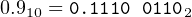(14.5)

which gives a round oﬀ error of

 0.910 −0.111001102 = 0.910 − 0.898437510 = 0.001562510 (14.6)

We note here that two’s complement also works correctly for storing negative fractional values. You are asked to show this in Exercise 14-2.

### 14.2 Fixed Point ints

In a ﬁxed point format, the storage area is divided into the integral part and the fractional part. The programmer must keep track of where the binary point is located. For example, we may decide to divide a 32-bit int in half and use the high-order 16 bits for the integral part and the low-order 16 bits for the fractional part.

My bank provides me with printed deposit slips that use this method. There are seven boxes for numerals. There is also a decimal point printed just to the left of the rightmost two boxes. Note that the decimal point does not occupy a box. That is, there are no digits allocated for the decimal point. So the bank assumes up to ﬁve decimal digits for the “dollars” part (rather optimistic), and the rightmost two decimal digits represent the “cents” part. The bank’s printing tells me how they have allocated the digits, but it is my responsibility to keep track of the location of the decimal point when ﬁlling in the digits.

One advantage of a ﬁxed point format is that integer instructions can be used for arithmetic computations. Of course, the programmer must be very careful to keep track of which bits are allocated for the integral part and which for the fractional part. And the range of possible values is restricted by the number of bits.

An example of using ints for ﬁxed point addition is shown in Listing 14.1.

1/*
3 * Adds two ruler measurements, to nearest 1/16th inch.
4 * Bob Plantz - 18 June 2009
5 */
6#include <stdio.h>
7
8int main(void)
9{
10    int x, y, fraction_part, sum;
11
12    printf("Enter first measurement, inches: ");
13    scanf("%d", &x);
14    x = x << 4;         /* shift to integral part of variable */
15    printf("                     sixteenths: ");
16    scanf("%d", &fraction_part);
17    x = x | (0xf & fraction_part);  /* add in fractional part */
18
19    printf("Enter second measurement, inches: ");
20    scanf("%d", &y);
21    y = y << 4;         /* shift to integral part of variable */
22    printf("                      sixteenths: ");
23    scanf("%d", &fraction_part);
24    y = y | (0xf & fraction_part);  /* add in fractional part */
25
26    sum = x + y;
27    printf("Their sum is %d and %d/16 inches\n",
28          (sum >> 4), (sum & 0xf));
29
30    return 0;
31}
Listing 14.1: Fixed point addition. The high-order 28 bits are used for the integral part, the low-order 4 for the fractional part.)

The numbers are input to the nearest 1/16th inch, so the programmer has allocated four bits for the fractional part. This leaves 28 bits for the integral part. After the integral part is read, the stored number must be shifted four bit positions to the left to put it in the high-order 28 bits. Then the fractional part (in number of sixteenths) is added into the low-order four bits with a simple bit-wise or operation. Printing the answer also requires some bit shifting and some masking to ﬁlter out the fractional part.

This is clearly a contrived example. A program using floats would work just as well and be somewhat easier to write. However, the program in Listing 14.1 uses integer instructions, which execute faster than ﬂoating point. The hardware issues have become less signiﬁcant in recent times. Modern CPUs use various parallelization schemes such that a mix of ﬂoating point and integer instructions may actually execute faster than only integer instructions. Fixed point arithmetic is often used in embedded applications where the CPU is small and may not have ﬂoating point capabilities.

### 14.3 Floating Point Format

The most important concept in this section is that ﬂoating point numbers are not real numbers.3 Real numbers include the continuum of all numbers from −∞ to +. You already understand that computers are ﬁnite, so there is certainly a limit on the largest values that can be represented. But the problem is much worse than simply a size limit.

As you will see in this section, ﬂoating point numbers comprise a very small subset of real numbers. There are signiﬁcant gaps between adjacent ﬂoating point numbers. These gaps can produce the following types of errors:

• Rounding — the number cannot be exactly represented in ﬂoating point.
• Absorption — a very small number gets lost when adding it to a large one.
• Cancellation — a very small number gets lost when subtracting it from a large one.

To make matters worse, these errors can occur in intermediate results, where they are very diﬃcult to debug.

The idea behind ﬂoating point formats is to think of numbers written in scientiﬁc format. This notation requires two numbers to completely specify a value — a signiﬁcand and an exponent. To review, a decimal number is written in scientiﬁc notation as a signiﬁcand times ten raised to an exponent. For example,

 1,024 = 1.024 × 103 (14.7) − 0.000089372 = −8.9372 × 10−5 (14.8)

Notice that the number is normalized such that only one digit appears to the left of the decimal point. The exponent of 10 is adjusted accordingly.

If we agree that each number is normalized and that we are working in base 10, then each ﬂoating point number is completely speciﬁed by three items:

1. The signiﬁcand.
2. The exponent.
3. The sign.

That is, in the above examples

• 1024, 3, and + represent 1.024 × 103 (The “+” is understood.)
• 89372, -5, and - represent 8.9372 × 105

The advantage of using a ﬂoating point format is that, for a given number of digits, we can represent a larger range of values. To illustrate this, consider a four-digit, unsigned decimal system. The range of integers that could be represented is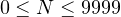Now, let’s allocate two digits for the signiﬁcand and two for the exponent. We will restrict our scheme to unsigned numbers, but we will allow negative exponents. So we will need to use one of the exponent digits to store the sign of the exponent. We will use 0 for positive and 1 for negative. For example, 3.9 × 104 would be stored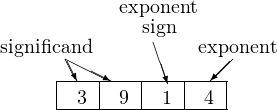where each box holds one decimal digit. Some other examples are:

 1000 represents 1.0 × 100 3702 represents 3.7 × 102 9316 represents 9.3 × 10−6

Our normalization scheme requires that there be a single non-zero digit to the left of the decimal point. We should also allow the special case of 0.0:

 0000 represents 0.0

A little thought shows that this scheme allows numbers in the rangeThat is, we have increased the range of possible values by a factor of 1014! However, it is important to realize that in both storage schemes, the integer and the ﬂoating point, we have exactly the same number of possible values — 104.

Although ﬂoating point formats can provide a much greater range of numbers, the distance between any two adjacent numbers depends upon the value of the exponent. Thus, ﬂoating point is generally less accurate than an integer representation, especially for large numbers.

To see how this works, let’s look at a plot of numbers (using our current scheme) in the rangeNotice that the larger numbers are further apart than the smaller ones. (See Exercise 14-7 after you read Section 14.4.)

Let us pick some numbers from this range and perform some addition.

 9111 represents 9.1 × 10−1 9311 represents 9.3 × 10−1

If we add these values, we get 0.91 + 0.93 = 1.84. Now we need to round oﬀ our “paper” addition in order to ﬁt this result into our current ﬂoating point scheme:

 1800 represents 1.8 × 100

On the other hand,

 9411 represents 9.4 × 10−1 9311 represents 9.3 × 10−1

and adding these values, we get 0.94 + 0.93 = 1.87. Rounding oﬀ, we get:

 1900 represents 1.9 × 100

So we see that starting with two values expressed to the nearest 1/100th, their sum is accurate only to the nearest 1/10.

To compare this with ﬁxed point arithmetic, we could use the same four digits to store 0.93 this way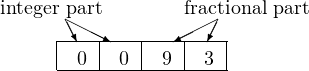It is clear that this storage scheme allows us to perform both additions (0.91 + 0.93 and 0.94 + 0.93) and store the results exactly.

These round oﬀ errors must be taken into account when performing ﬂoating point arithmetic. In particular, the errors can occur in intermediate results when doing even moderately complex computations, where they are very diﬃcult to detect.

### 14.4 IEEE 754

Speciﬁc ﬂoating point formats involve trade-oﬀs between re4solution, round oﬀ errors, size, and range. The most commonly used formats are the IEEE 754.4 They range in size from four to sixteen bytes. The most common sizes used in C/C++ are floats (4 bytes) and doubles (8 bytes). The x86 processor performs ﬂoating point computations using an extended 10-byte format. The results are rounded to 4-byte mode if the programmer uses the float data type or 8-byte mode for the double data type.

In the IEEE 754 4-byte format, one bit is used for the sign, eight for the exponent, and twenty-three for the signiﬁcand. The IEEE 754 8-byte format speciﬁes one bit for the sign, eleven for the exponent, and ﬁfty-two for the signiﬁcand.

In this section we describe the 4-byte format in order to save ourselves (hand) computation eﬀort. The goal is to get a feel for the limitations of ﬂoating point formats. The normalized form of the number in binary is given by Equation 14.9.(14.9)

 where: s is the sign bit f is the 23-bit fractional part e is the 8-bit exponent

The bit patterns for floats and doubles are arranged as shown in Figure 14.1.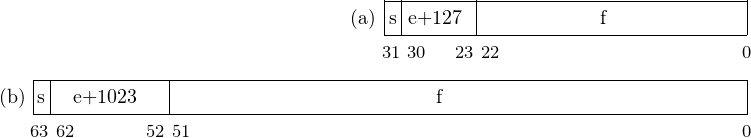Figure 14.1: IEEE 754 bit patterns. (a) Float. (b) Double.

As in decimal, the exponent is adjusted such that there is only one non-zero digit to the left of the binary point. In binary, though, this digit is always one. Since it is always one, it need not be stored. Only the fractional part of the normalized value needs to be stored as the signiﬁcand. This adds one bit to the signiﬁcance of the fractional part. The integer part (one) that is not stored is sometimes called the hidden bit.

The sign bit, s, refers to the number. Another mechanism is used to represent the sign of the exponent, e. Your ﬁrst thought is probably to use two’s complement. However, the IEEE format was developed in the 1970s, when ﬂoating point computations took a lot of CPU time. Many algorithms depend upon only the comparison of two numbers, and the computer scientists of the day realized that a format that allowed integer comparison instructions would result in faster execution times. So they decided to store a biased exponent as an unsigned int. The amount of the bias is one-half the range allocated for the exponent. In the case of an 8-bit exponent, the bias amount is 127.

Example 14-a ____________________________________________________________________________________________________

Show how 97.8125 is stored in 32-bit IEEE 754 binary format.

Solution:

First, convert the number to binary.

 97.812510 = 1100001.11012 = (−1)0 ×1100001.1101× 20

Adjust the exponent to obtain the normalized form.Compute s, e+127, and f.

 s = 0 e + 127 = 6 + 127 = 133 = 100001012 f = 10000111010000000000000

Finally, use Figure 14.1 to place the bit patterns. (Remember that the hidden bit is not stored; it is understood to be there.)

 97.8125 is stored as 0 10000101 100001110100000000000002 or 42c3a00016
_______________________________________________________________________________

Example 14-b ____________________________________________________________________________________________________

Using IEEE 754 32-bit format, what decimal number does the bit pattern 3e40000016 represent?

Solution:

First, convert the hexadecimal to binary, using spaces suggested by Figure 14.1.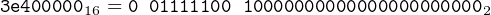Now compute the values of s, e, and f.

 s = 0 e + 127 = 011111002 = 12410 e = −310 f = 10000000000000000000000

Finally, plug these values into Equation 14.9. (Remember to add the hidden bit.)

 (−1)0 ×1.100…00× 2−3 = (−1)0 ×0.0011× 20 = 0.187510
__________________________________________________________________________________________________________________________________________________________

Example 14-c ____________________________________________________________________________________________________

Using IEEE 754 32-bit format, what decimal number would the bit pattern 0000000016 represent? (The speciﬁcation states that it is an exception to the format and is deﬁned to represent 0.0. This example provides some motivation for this exception.)

Solution:

The conversion to binary is trivial. Computing the values of s, e, and f.

 s = 0 e + 127 = 000000002 e = −12710 f = 00000000000000000000000

Finally, plug these values into Equation 14.9. (Remember to add the hidden bit.)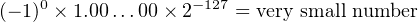__________________________________________________________________________________

This last example illustrates a problem with the hidden bit — there is no way to represent zero. To address this issue, the IEEE 754 standard has several special cases.

• Zero — all the bits in the exponent and signiﬁcand are zero. Notice that this allows for -0.0 and +0.0, although (-0.0 == +0.0) computes to true.
• Denormalized — all the bits in the exponent are zero. In this case there is no hidden bit. Zero can be thought of as a special case of denormalized.
• Inﬁnity — all the bits in the exponent are one, and all the bits in the signiﬁcand are zero. The sign bit allows for −∞ and +.
• NaN — all the bits in the exponent are one, and the signiﬁcand is non-zero. This is used when the results of an operation are undeﬁned. For example, ±nonzero ÷ 0.0 yields inﬁnity, but ±0.0 ÷ ±0.0 yields NaN.

### 14.5 Floating Point Hardware

Until the introduction of the Intel 486DX in4 April 1989, the x87 Floating Point Unit was on a separate chip. It is now included on the CPU chip although it uses a somewhat diﬀerent execution architecture than the Integer Unit in the CPU.

In 1997 Intel added MMX(Multimedia Extensions) to their processors, which includes instructions that process multiple data values with a single instruction (SIMD). Operations on single data items are called scalar operations, while those on multiple data items in parallel are called vector operations. Vector operations are useful for many multi-media and scientiﬁc applications. In this book we will discuss only scalar ﬂoating point operations. Originally, MMX only performed integer computations. But in 1998 AMD added the 3DNow!extension to MMX, which includes ﬂoating point instructions. Intel soon followed suit.

Intel then introduced SSE (Streaming SIMD Extension) on the Pentium III in 1999. Several versions have evolved over the years — SSE, SSE2, SSE3, and SSE4A — as of this writing. There are instructions for performing both integer and ﬂoating point operations on both scalar and vector values.

The x86-64 architecture includes three sets of instructions for working with ﬂoating point values:

• SSE2 instructions operate on 32-bit or 64-bit values.5 Four 32-bit values or two 64-bit values can be processed simultaneously.
• x87 Floating Point Unit instructions operate on 80-bit values.
• 3DNow! instructions operate on two 32-bit values.

All three ﬂoating point instruction sets include a wide variety of instructions to perform the following operations:

• Move data from memory to a register, from a register to memory, and from a register to another register.
• Convert data from integer to ﬂoating point, and from ﬂoating point to integer formats.
• Perform the usual add, subtract, multiply, and divide arithmetic operations. They also provide square root instructions.
• Compare two values.
• Perform the usual and, or, and xor logical operations.

In addition, the x87 includes instructions for transcendental functions — sine, cosine, tangent, and arc tangent, and logarithm functions.

We will not cover all the instructions in this book. The following subsections provide an introduction to how each of the three sets of instructions is used. See the manuals  and  for details.

#### 14.5.1 SSE2 Floating Point

Most of the SSE2 instructions operate on multiple data items simultaneously — single instruction, multiple data (SIMD). There are SSE2 instructions for both integer and ﬂoating point operations. Integer instructions operate on up to sixteen 8-bit, eight 16-bit, four 32-bit, two 64-bit, or one 128-bit integers at a time. Vector ﬂoating point instructions operate on all four 32-bit or both 64-bit ﬂoats in a register simultaneously. Each data item is treated independently. These instructions are useful for algorithms that do things like process arrays. One SSE2 instruction can operate on several array elements in parallel, resulting in considerable speed gains. Such algorithms are common in multi-media and scientiﬁc applications.

In this book we will only consider some of the scalar ﬂoating-point instructions, which operate on only single data items. The SSE2 instructions are the preferred ﬂoating-point implementation in 64-bit mode. These instructions operate on either 32-bit (single-precision) or 64-bit (double-precision) values. The scalar instructions operate on only the low-order portion of the 128-bit xmm registers, with the high-order 64 or 96 bits remaining unchanged.

SSE includes a 32-bit MXCSR register that has ﬂags for handling ﬂoating-point arithmetic errors. These ﬂags are shown in Table 14.1.

 bits mnemonic meaning default 31 – 18 – reserved 17 MM Misaligned Exception Mask 0 16 – reserved 15 FZ Flush-toZero for Masked Underﬂow 0 14 – 13 RC Floating-Point Rounding Control 00 12 PM Precision Exception Mask 1 11 UM Underﬂow Exception Mask 1 10 OM Overﬂow Exception Mask 1 9 ZM Zero-Divide Exception Mask 1 8 DM Denormalized-Operand Exception Mask 1 7 IM Invalid-Operation Exception Mask 1 6 DAZ Denormals Are Zero 0 5 PE Precision Exception 0 4 UE Underﬂow Exception 4 0 3 OE Overﬂow Exception 0 2 ZE Zero-Divide Exception 1 1 DE Denormalized-Operand Exce4ption 0 0 IE Invalid-Operation Exception 0

Table 14.1: MXCSR status register.

SSE instructions that perform arithmetic operations and the SSE compare instructions also aﬀect the status ﬂags in the rflags register. Thus the regular conditional jump instructions (Section 10.1.2, page 676) are used to control program ﬂow based on ﬂoating-point computations.

The instruction mnemonics used by the gnu assembler are mostly the same as given in the manuals,  and . Since they are quite descriptive with respect to operand sizes, a size letter is not appended to the mnemonic, except when one of the operands is in memory and the size is ambiguous. Of course, the operand order used by the gnu assembler is still reversed compared to the manufacturers’ manuals, and the register names are preﬁxed with the “%” character.

A very important set of instructions provided for working with ﬂoating point values are those to convert between integer and ﬂoating point formats. The scalar conversion SSE2 instructions are shown in Table 14.2.

 mnemonic source destination meaning cvtsd2si xmm reg. 32-bit integer convert scalar 64-bit or mem. reg. ﬂoat to 32-bit integer cvtsd2ss xmm reg. xmm reg. or convert scalar 64-bit mem. ﬂoat to 32-bit ﬂoat cvtsi2sd integer reg. xmm reg. convert 32-bit integer or mem. to scalar 64-bit ﬂoat cvtsi2sdq integer reg. xmm reg. convert 64-bit integer or mem. to scalar 64-bit ﬂoat cvtsi2ss integer reg. xmm reg. convert 32-bit integer or mem. to scalar 32-bit ﬂoat cvtsi2ssq integer reg. xmm reg. convert 64-bit integer or mem. to scalar 32-bit ﬂoat cvtss2sd xmm reg. another xmm reg. convert scalar 32-bit or mem. ﬂoat to 64-bit ﬂoat cvtss2si xmm reg. 32-bit integer convert scalar 32-bit or mem. reg. ﬂoat to 32-bit integer cvtss2siq xmm reg. 64-bit integer convert scalar 32-bit or mem. reg. ﬂoat to 64-bit integer

Table 14.2: SSE scalar ﬂoating point conversion instructions. Source and destination xmm registers must be diﬀerent. The low-order portion of the xmm register is used. When reading from or writing to memory, the “q” suﬃx is used to designate a 64-bit value.

Data movement and arithmetic instructions must distinguish between scalar and vector operations on values in the xmm registers. The low-order portion of the register is used for scalar operations. Vector operations are performed on multiple data values packed into a single register. See Table 14.3 for a sampling of SSE2 data movement and arithmetic instructions.

 mnemonic source destination meaning addps xmm reg. or mem. xmm reg. add packed 32-bit ﬂoats addpd xmm reg. or mem. xmm reg. add packed 64-bit ﬂoats addss xmm reg. or mem. xmm reg. add scalar 32-bit ﬂoats addsd xmm reg. or mem. xmm reg. add scalar 64-bit ﬂoats divps xmm reg. or mem. xmm reg. divide packed 32-bit ﬂoats divpd xmm reg. or mem. xmm reg. divide packed 64-bit ﬂoats divss xmm reg. or mem. xmm reg. divide scalar 32-bit ﬂoats divsd xmm reg. or mem. xmm reg. divide scalar 64-bit ﬂoats movss xmm reg. or mem. xmm reg. move scalar 32-bit ﬂoat movss xmm reg. xmm reg. or mem. move scalar 32-bit ﬂoat movsd xmm reg. or mem. xmm reg. move scalar 64-bit ﬂoat movsd xmm reg. xmm reg. or mem. move scalar 64-bit ﬂoat mulps xmm reg. or mem. xmm reg. multiply packed 32-bit ﬂoats mulpd xmm reg. or mem. xmm reg. multiply packed 64-bit ﬂoats mulss xmm reg. or mem. xmm reg. multiply scalar 32-bit ﬂoats mulsd xmm reg. or mem. xmm reg. multiply scalar 64-bit ﬂoats subps xmm reg. or mem. xmm reg. subtract packed 32-bit ﬂoats subpd xmm reg. or mem. xmm reg. subtract packed 64-bit ﬂoats subss xmm reg. or mem. xmm reg. subtract scalar 32-bit ﬂoats subsd xmm reg. or mem. xmm reg. subtract scalar 64-bit ﬂoats

Table 14.3: Some SSE ﬂoating point arithmetic and data movement instructions. Source and destination xmm registers must be diﬀerent. Scalar instructions use the low-order portion of the xmm registers.

Notice that the code for the basic operation is followed by a “p” or “s” for “packed” or “scalar.” This character is then followed by a “d” or “s” for “double” (64-bit) or “single” (32-bit) data item.

We will use the program in Listing 14.2 to illustrate a few ﬂoating point operations.

1/*
2 * frac2float.c
3 * Converts fraction to floating point.
4 * Bob Plantz - 18 June 2009
5 */
6
7#include <stdio.h>
8
9int main(void)
10{
11    int x, y;
12    double z;
13
14    printf("Enter two integers: ");
15    scanf("%i %i", &x, &y);
16    z = (double)x / y;
17    printf("%i / %i = %lf\n", x, y, z);
18    return 0;
19 }
Listing 14.2: Converting a fraction to a ﬂoat.

Compiling this program in 64-bit mode produced the assembly language in Listing 14.3.

1        .file  "frac2float.c"
2        .section      .rodata
3.LC0:
4        .string"Enter two integers: "
5.LC1:
6        .string"%i %i"
7.LC2:
8        .string"%i / %i = %lf\n"
9        .text
10        .globlmain
11        .type  main, @function
12main:
13        pushq  %rbp
14        movq  %rsp, %rbp
15        subq  \$32, %rsp
16        movl  \$.LC0, %edi
17        movl  \$0, %eax
18        call  printf
19        leaq  -12(%rbp), %rdx     # address of y
20        leaq  -16(%rbp), %rax     # address of x
21        movq  %rax, %rsi
22        movl  \$.LC1, %edi
23        movl  \$0, %eax            # no xmm arguments
24        call  __isoc99_scanf
25        movl  -16(%rbp), %eax     # load x
26        cvtsi2sd      %eax, %xmm0 # convert to double
27        movl  -12(%rbp), %eax     # load y
28        cvtsi2sd      %eax, %xmm1 # convert to double
29        divsd  %xmm1, %xmm0        # (double)x / (double)y
30        movsd  %xmm0, -8(%rbp)     # store z
31        movl  -12(%rbp), %edx     # load y
32        movl  -16(%rbp), %ecx     # load x
33        movq  -8(%rbp), %rax
34        movq  %rax, -24(%rbp)
35        movsd  -24(%rbp), %xmm0    # load z
36        movl  %ecx, %esi          # x to correct register
37        movl  \$.LC2, %edi
38        movl  \$1, %eax            # one xmm argument (in xmm0)
39        call  printf
40        movl  \$0, %eax
41        leave
42        ret
43        .size  main, .-main
44        .ident"GCC: (Ubuntu/Linaro 4.7.0-7ubuntu3) 4.7.0"
45        .section      .note.GNU-stack,"",@progbits
Listing 14.3: Converting a fraction to a ﬂoat (gcc assembly language, 64-bit).

Before the division is performed, both integers must be converted to ﬂoating point. This takes place on lines 25 – 28:

25        movl  -16(%rbp), %eax     # load x
26        cvtsi2sd      %eax, %xmm0 # convert to double
27        movl  -12(%rbp), %eax     # load y
28        cvtsi2sd      %eax, %xmm1 # convert to double

The cvtsi2sd instruction on lines 26 and 28 converts a signed integer to a scalar double-precision ﬂoating point value. The signed integer can be either 32 or 64 bits and can be located in a general purpose register or in memory. The double-precision ﬂoat will be stored in the low-order 64 bits of the speciﬁed xmmn register. The high-order 64 bits to the xmmn register are not changed.

The division on line 29 leaves the result in the low-order 64 bits of xmm0, which is then stored in z:

29        divsd  %xmm1, %xmm0        # (double)x / (double)y
30        movsd  %xmm0, -8(%rbp)     # store z

The movapd instruction moves the entire 128 bits, and the movsd instruction moves only the low-order 64 bits.

The ﬂoating point arguments are passed in the registers xmm0, xmm1, …, xmm15 in left-to-right order. So the value of z is loaded into the (xmm0) register for passing to the printf function, and the number of ﬂoating point values passed to it must be stored in eax:

35        movsd  -24(%rbp), %xmm0    # load z
36        movl  %ecx, %esi          # x to correct register
37        movl  \$.LC2, %edi
38        movl  \$1, %eax            # one xmm argument (in xmm0)
39        call  printf

#### 14.5.2 x87 Floating Point Unit

The x87 FPU has eight 80-bit data registers, its own status register, and its own stack pointer. Floating point values are stored in the ﬂoating point data registers in an extended format.:

• bit 79 is the sign bit: 0 for positive, 1 for negative.
• bits 78 – 64 are for the exponent: 2’s complement, biased by 16383.
• bit 63 is the integer: 1 for normalized.
• bits 62 – 0 are for the fraction.

So there are 64 bits (63 – 0) for the signiﬁcand. Since there is no hidden bit in the extended format, one of these bits, bit 63, is required for the integer part.

Example 14-d ____________________________________________________________________________________________________

Show how 97.8125 is stored in 80-bit extended IEEE 754 binary format.

Solution:

First, convert the number to binary.

 97.812510 = 1100001.11012 (−1)0 ×1100001.1101× 20

Adjust the exponent to obtain the normalized form.Compute s, e+16383.

 s = 0 e + 16383 = 6 + 16383 = 16389 = 1000000000001012

Filling in the bit patterns as speciﬁed above:

 97.8125 is stored as 0 100000000000101 1 1000011101000000000000…02 or 4005c3a000000000000016

Compare this with the 32-bit format in Example 14-a above.

__________________________________________________________________________________

The 16-bit Floating Point Unit Status Word register shows the results of ﬂoating point operations. The meaning of each bit is shown in Table 14.4.

 bit number mnemonic meaning 0 IE invalid operation 1 DE denormalized operation 2 ZE zero divide 3 OE overﬂow 4 UE underﬂow 5 PE precision 6 SF stack fault 7 ES error summary status 8 C0 condition code 0 9 C1 condition code 1 10 C2 condition code 2 11 – 13 TOP top of stack 14 C3 condition code 3 15 B FPU busy

Table 14.4: x87 Status Word.

Figure 14.2 shows a pictorial representation of the x87 ﬂoating point registers. The absolute locations are named fpr0, fpr1,…,fpr7 in this ﬁgure.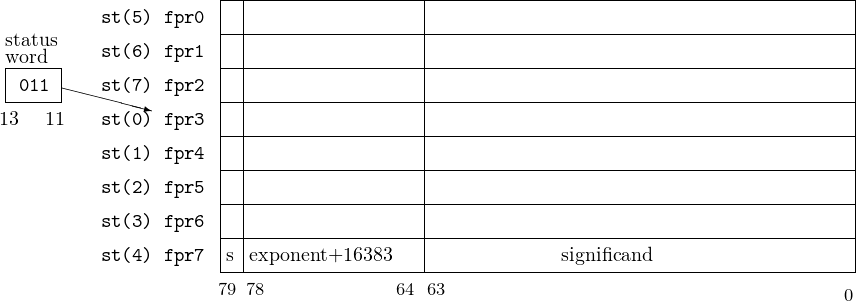Figure 14.2: x87 ﬂoating point register stack. The fpri represent the absolute locations. The st(j) are the stack names, which are used by the instructions. In this example the top of the stack is at fpr3, as shown in bits 13 – 11 of the x87 status register.

The ﬂoating point registers are accessed by program instructions as a stack with st(0) being the register at the top of the stack. It “grows” from higher number registers to lower. The TOP ﬁeld (bits 13 – 11) in the FPU Status Word holds the (absolute) register number that is currently the top of the stack. If the stack is full, i.e., fpr0 is the top of the stack, a push causes the TOP ﬁeld to roll over, and the next item goes into register fpr7. (The value that was in fpr7 is lost.)

The instructions that read data from memory automatically push the value onto the top of the register stack. Arithmetic instructions are provided that operate on the value(s) on the top of the stack. For example, the faddp instruction adds the two values on the top of the stack and leaves their sum on the top. The stack has one less value on it. The original two values are gone.

Many ﬂoating point instructions allow the programmer to access any of the ﬂoating point registers, %st(i), where i = 0…7, relative to the top of the stack. Fortunately, the programmer does not need to keep track of where the top is. When using this format, %st(i) refers to the ith register from the top of the stack. For example, if fpr3 is the current top of the stack, the instruction

will add the value in the fpr5 register to the value in the fpr3 register, leaving the result in the fpr3 register.

Table 14.5 provides some examples of the ﬂoating point instruction set. Notice that the instructions that deal only with the ﬂoating point register stack do not use the size suﬃx letter, s.

 mnemonic source destination meaning fadds memﬂoat add memﬂoat to st(0) faddp add st(0) to st(1) and pop register stack fchs change sign of st(0) fcoms memﬂoat compare st(0) with memﬂoat fcomp compare st(0) with st(1) and pop register stack fcos replace st(0) with its cosine fdivs memﬂoat divide st(0) by memﬂoat fdivp divide st(0) by st(1), store result in st(1), and pop register stack filds memint convert integer at memint to 80-bit ﬂoat and push onto register stack fists memint convert 80-bit ﬂoat at st(0) to int and store at memint flds memﬂoat convert ﬂoat at memint to 80-bit ﬂoat and push onto register stack fmuls memﬂoat multiply st(0) by memﬂoat fmulp multiply st(0) by st(1), store result in st(1), and pop register stack fsin replace st(0) with its sine fsqrt replace st(0) with its square root fsts memint convert 80-bit ﬂoat at st(0) to s size ﬂoat and store at memint fsubs memﬂoat subtract memﬂoat from st(0) fsubp subtract st(0) from st(1) and pop register stack s = s, l, t

Table 14.5: A sampling of x87 ﬂoating point instructions. Size characters are: s = 32-bit, l = 64-bit, t = 80-bit.

To avoid ambiguity the gnu assembler requires a single letter suﬃx on the ﬂoating point instructions that access memory. The suﬃxes are:

 ’s’ for single precision – 32-bit ’l’ for long (or double) precision – 64-bit ’t’ for ten-byte – 80-bit

Most of the ﬂoating point instructions have several variants. See  and  for details. In general,

• Data cannot be moved directly between the integer and ﬂoating point registers. Only data stored in memory or another ﬂoating point register can be pushed onto the ﬂoating point register stack.
• st(0) is always involved when performing ﬂoating point arithmetic.
• Many ﬂoating point instructions have a pop variant. The mnemonic includes a ‘p’ after the basic mnemonic, immediately before the size character. For example,
fistl    someplace(%ebp)

converts the 80-bit ﬂoating point number in st(0) to a 32-bit integer and stores it at the speciﬁed memory location. Using the pop variant,

fistpl   someplace(%ebp)

does the same thing but also pops one from the ﬂoating point register stack.

Compiling the fraction conversion program of Listing 14.2 in 32-bit mode shows (Listing 14.4) that the compiler uses the x87 ﬂoating-point instructions. This ensures backward compatibility since the x86-32 architecture does not need to include SSE instructions.

1        .file  "frac2float.c"
2        .section      .rodata
3.LC0:
4        .string"Enter two integers: "
5.LC1:
6        .string"%i %i"
7.LC2:
8        .string"%i / %i = %lf\n"
9        .text
10        .globlmain
11        .type  main, @function
12main:
13        pushl  %ebp
14        movl  %esp, %ebp
15        andl  \$-16, %esp
16        subl  \$64, %esp
17        movl  \$.LC0, (%esp)
18        call  printf
19        leal  52(%esp), %eax   # address of x
20        movl  %eax, 8(%esp)
21        leal  48(%esp), %eax   # address of y
22        movl  %eax, 4(%esp)
23        movl  \$.LC1, (%esp)
24        call  __isoc99_scanf
25        movl  48(%esp), %eax   # load y
26        movl  %eax, 44(%esp)   # needs to be in memory to
27        fildl  44(%esp)         # convert to 80-bit float and push
28        movl  52(%esp), %eax   # load x
29        movl  %eax, 44(%esp)
30        fildl  44(%esp)         # convert to 80-bit float and push
31        fdivrp%st, %st(1)       # (long double)x / (long double) y
32        fstpl  56(%esp)         # store z
33        movl  52(%esp), %edx   # load x
34        movl  48(%esp), %eax   # load y
35        fldl  56(%esp)         # load z
36        fstpl  12(%esp)         # put z on call stack
37        movl  %edx, 8(%esp)    # put x on call stack
38        movl  %eax, 4(%esp)    # put y on call stack
39        movl  \$.LC2, (%esp)
40        call  printf
41        movl  \$0, %eax
42        leave
43        ret
44        .size  main, .-main
45        .ident"GCC: (Ubuntu/Linaro 4.7.0-7ubuntu3) 4.7.0"
46        .section      .note.GNU-stack,"",@progbits
Listing 14.4: Converting a fraction to a ﬂoat (gcc assembly language, 32-bit).

19        leal  52(%esp), %eax   # address of x
20        movl  %eax, 8(%esp)
21        leal  48(%esp), %eax   # address of y
22        movl  %eax, 4(%esp)
23        movl  \$.LC1, (%esp)
24        call  __isoc99_scanf

Rather than actually push the arguments onto the stack, enough space was allocated on the stack (line 15) to directly store the values in the location where they would be if they had been pushed there. This is more eﬃcient that pushing each argument.

Casting an int to a float requires a conversion in the storage format. This conversion is done by the x87 FPU as an integer is pushed onto the ﬂoating point register stack using the fildl instruction. This conversion can only be done to an integer that is stored in memory. The compiler uses a location on the call stack to temporarily store each integer so it can be converted:

25        movl  48(%esp), %eax   # load y
26        movl  %eax, 44(%esp)   # needs to be in memory to
27        fildl  44(%esp)         # convert to 80-bit float and push
28        movl  52(%esp), %eax   # load x
29        movl  %eax, 44(%esp)
30        fildl  44(%esp)         # convert to 80-bit float and push

Each of the fildl instructions on lines 27 and 30 converts a 32-bit integer to an 80-bit ﬂoat and pushes the ﬂoat onto the x87 register stack. At this point the ﬂoating-point equivalent of x is at the top of the stack, and the ﬂoating-point equivalent of y is immediately below it. Then the ﬂoating-point division instruction:

31        fdivrp%st, %st(1)       # (long double)x / (long double) y

divides the number at st(0) (the (0) can be omitted) by the number at st(1) and pops the x87 register stack so that the result is now at the top of the stack.

Finally, the fstpl instruction is used to pop the value oﬀ the top of the x87 register stack and store it in memory — at its proper location on the call stack. The “l” suﬃx indicates that 64 bits of memory should be used for storing the ﬂoating-point value. So the 80-bit value on the top of the x87 register stack is rounded to 64 bits as it is stored in memory. The other three arguments are also stored on the call stack.

32        fstpl  56(%esp)         # store z
33        movl  52(%esp), %edx   # load x
34        movl  48(%esp), %eax   # load y
35        fldl  56(%esp)         # load z
36        fstpl  12(%esp)         # put z on call stack
37        movl  %edx, 8(%esp)    # put x on call stack
38        movl  %eax, 4(%esp)    # put y on call stack

Note that the 32-bit version of printf does not receive arguments in registers, so eax is not used.

#### 14.5.3 3DNow! Floating Point

The 3DNow! instructions use the low-order 64 bits in the same physical registers at the x87. These 64-bit portions are named mmx0, mmx1,…, mmx7. They are used as ﬁxed register, not in a stack conﬁguration. Execution of a 3DNow! instruction changes the TOP ﬁeld (bits 13 – 11) in the MXCSR status register, so the top of stack is lost for any subsequent x87 instructions. The bottom line is that x87 and 3DNow! instructions cannot be used simultaneously.

Another limitation of the 3DNow! instructions set is that it only handles 32-bit ﬂoating point.

These limitations of the 3DNow! instruction set make it essentially obsolete in the x86-64 architecture, so it will not be discussed further in this book.

Beginning programmers often see ﬂoating point arithmetic as more accurate than integer. It is true that even adding two very large integers can cause overﬂow. Multiplication makes it even more likely that the result will be very large and, thus, overﬂow. And when used with two integers, the / operator in C/C++ causes the fractional part to be lost. However, as you have seen in this chapter, ﬂoating point representations have their own set of inaccuracies.

Arithmetically accurate results require a thorough analysis of your algorithm. Some points to consider:

• Try to scale the data such that integer arithmetic can be used.
• All ﬂoating point computations are performed in 80-bit extended format. So there is no processing speed improvement from using floats instead of doubles.
• Try to arrange the order of computations so that similarly sized numbers are added or subtracted.
• Avoid complex arithmetic statements, which may obscure incorrect intermediate results.
• Choose test data that “stresses” your algorithm. For example, 0.00390625 can be stored exactly in eight bits, but 0.1 has no exact binary equivalent.

### 14.7 Instructions Introduced Thus Far

This summary shows the assembly language instructions introduced thus far in the book. The page number where the instruction is explained in more detail, which may be in a subsequent chapter, is also given. This book provides only an introduction to the usage of each instruction. You need to consult the manuals (, ) in order to learn all the possible uses of the instructions.

#### 14.7.1 Instructions

 data movement: opcode source destination action page cbtw convert byte to word, al → ax 696 cwtl convert word to long, ax → eax 696 cltq convert long to quad, eax → rax 696 cwtd convert word to long, ax → dx:ax 786 cltd convert long to quad, eax → edx:eax 786 cqto convert quad to octuple, rax → rdx:rax 786 cmovcc %reg/mem %reg conditional move 706 movs \$imm/%reg %reg/mem move 506 movs mem %reg move 506 movsss \$imm/%reg %reg/mem move, sign extend 693 movzss \$imm/%reg %reg/mem move, zero extend 693 popw %reg/mem pop from stack 566 pushw \$imm/%reg/mem push onto stack 566 s = b, w, l, q; w = l, q; cc = condition codes

 arithmetic/logic: opcode source destination action page adds \$imm/%reg %reg/mem add 607 adds mem %reg add 607 ands \$imm/%reg %reg/mem bit-wise and 747 ands mem %reg bit-wise and 747 cmps \$imm/%reg %reg/mem compare 676 cmps mem %reg compare 676 decs %reg/mem decrement 699 divs %reg/mem unsigned divide 777 idivs %reg/mem signed divide 784 imuls %reg/mem signed multiply 775 incs %reg/mem increment 698 leaw mem %reg load eﬀective address 579 muls %reg/mem unsigned multiply 769 negs %reg/mem negate 789 ors \$imm/%reg %reg/mem bit-wise inclusive or 747 ors mem %reg bit-wise inclusive or 747 sals \$imm/%cl %reg/mem shift arithmetic left 756 sars \$imm/%cl %reg/mem shift arithmetic right 751 shls \$imm/%cl %reg/mem shift left 756 shrs \$imm/%cl %reg/mem shift right 751 subs \$imm/%reg %reg/mem subtract 612 subs mem %reg subtract 612 tests \$imm/%reg %reg/mem test bits 676 tests mem %reg test bits 676 xors \$imm/%reg %reg/mem bit-wise exclusive or 747 xors mem %reg bit-wise exclusive or 747 s = b, w, l, q; w = l, q

 program ﬂow control: opcode location action page call label call function 546 ja label jump above (unsigned) 683 jae label jump above/equal (unsigned) 683 jb label jump below (unsigned) 683 jbe label jump below/equal (unsigned) 683 je label jump equal 679 jg label jump greater than (signed) 686 jge label jump greater than/equal (signed) 686 jl label jump less than (signed) 686 jle label jump less than/equal (signed) 686 jmp label jump 691 jne label jump not equal 679 jno label jump no overﬂow 679 jcc label jump on condition codes 679 leave undo stack frame 580 ret return from function 583 syscall call kernel function 587 cc = condition codes

 x87 ﬂoating point: opcode source destination action page fadds memﬂoat add 859 faddp add/pop 859 fchs change sign 859 fcoms memﬂoat compare 859 fcomp compare/pop 859 fcos cosine 859 fdivs memﬂoat divide 859 fdivp divide/pop 859 filds memint load integer 859 fists memint store integer 859 flds memﬂoat load ﬂoating point 859 fmuls memﬂoat multiply 859 fmulp multiply/pop 859 fsin sine 859 fsqrt square root 859 fsts memint ﬂoating point store 859 fsubs memﬂoat subtract 859 fsubp subtract/pop 859 s = s, l, t

 SSE ﬂoating point conversion: opcode source destination action page cvtsd2si %xmmreg/mem %reg scalar double to signed integer 845 cvtsd2ss %xmmreg %xmmreg/%reg scalar double to single ﬂoat 845 cvtsi2sd %reg %xmmreg/mem signed integer to scalar double 845 cvtsi2sdq %reg %xmmreg/mem signed integer to scalar double 845 cvtsi2ss %reg %xmmreg/mem signed integer to scalar single 845 cvtsi2ssq %reg %xmmreg/mem signed integer to scalar single 845 cvtss2sd %xmmreg %xmmreg/mem scalar single to scalar double 845 cvtss2si %xmmreg/mem %reg scalar single to signed integer 845 cvtss2siq %xmmreg/mem %reg scalar single to signed integer 845

__________________________________________________________
 register direct: The data value is located in a CPU register. syntax: name of the register with a “%” preﬁx. example: movl    %eax, %ebx immediate data: The data value is located immediately after the instruction. Source operand only. syntax: data value with a “\$” preﬁx. example: movl    \$0xabcd1234, %ebx base register plus oﬀset: The data value is located in memory. The address of the memory location is the sum of a value in a base register plus an oﬀset value. syntax: use the name of the register with parentheses around the name and the oﬀset value immediately before the left parenthesis. example: movl    \$0xaabbccdd, 12(%eax) rip-relative: The target is a memory address determined by adding an oﬀset to the current address in the rip register. syntax: a programmer-deﬁned label example: je     somePlace indexed: The data value is located in memory. The address of the memory location is the sum of the value in the base_register plus scale times the value in the index_register, plus the oﬀset. syntax: place parentheses around the comma separated list (base_register, index_register, scale) and preface it with the oﬀset. example: movl    \$0x6789cdef, -16(%edx, %eax, 4)

### 14.8 Exercises

14-1

14.1) Develop an algorithm for converting decimal fractions to binary. Hint: Multiply both sides of Equation 14.1 by two.

14-2

14.1) Show that two’s complement works correctly for fractional values. What is the decimal range of 8-bit, two’s complement fractional values? Hint: +0.5 does not exist, but -0.5 does.

14-3

14.3) Copy the following program and run it:

1/*
2 * exer14_3.c
3 * Use float for Loop Control Variable?
4 * Bob Plantz - 18 June 2009
5 */
6
7#include <stdio.h>
8
9int main()
10{
11    float number;
12    int counter = 20;
13
14    number = 0.5;
15    while ((number != 0.0) && (counter > 0))
16    {
17        printf("number = %.10f and counter = %i\n", number, counter);
18
19        number -= 0.1;
20        counter -= 1;
21    }
22
23    return 0;
24}
Listing 14.5: Use ﬂoat for Loop Control Variable?

Explain the behavior. What happens if you change the decrement of number from 0.1 to 0.0625? Explain.

14-4

14.3 §14.4) Copy the following program and run it:

1/*
2 * exer14_3.c
3 * Are floats accurate?
4 * Bob Plantz - 18 June 2009
5 */
6
7#include <stdio.h>
8
9int main()
10{
11    float fNumber = 2147483646.0;
12    int iNumber = 2147483646;
13
14    printf(" Before adding the float is %f\n", fNumber);
15    printf("         and the integer is %i\n\n", iNumber);
16    fNumber += 1.0;
17    iNumber += 1;
18    printf("After adding 1 the float is %f\n", fNumber);
19    printf("         and the integer is %i\n", iNumber);
20
21    return 0;
22}
Listing 14.6: Are ﬂoats accurate?

Explain the behavior. What is the maximum value of fNumber such that adding 1.0 to it works?

14-5

14.4) Convert the following decimal numbers to 32-bit IEEE 754 format by hand:

a) 1.0 b) -0.1 c) 2005.0 d) 0.00390625 e) -3125.3125 f) 0.33 g) -0.67 h) 3.14

14-6

14.4) Convert the following 32-bit IEEE 754 bit patterns to decimal.

a) 4000 0000 b) bf80 0000 c) 3d80 0000 d) c180 4000 e) 42c8 1000 f) 3f99 999a g) 42f6 e666 h) c259 48b4

14-7

14.4) Show that half the floats (in 32-bit IEEE 754 format) are between -2.0 and +2.0.

14-8

14.5) The following C program

1/*
2 * casting.c
3 * Casts two integers to floats and adds them.
4 * Bob Plantz - 18 June 2009
5 */
6
7#include <stdio.h>
8
9int main()
10{
11    int x;
12    double y, z;
13
14    printf("Enter an integer: ");
15    scanf("%i", &x);
16    y = 1.23;
17    z = (double)x + y;
18    printf("%i + %lf = %lf\n", x, y, z);
19
20    return 0;
21}
Listing 14.7: Casting integer to ﬂoat in C.

was compiled with the -S option to produce

1        .file  "casting.c"
2        .section      .rodata
3.LC0:
4        .string"Enter an integer: "
5.LC1:
6        .string"%i"
7.LC3:
8        .string"%i + %lf = %lf\n"
9        .text
10        .globlmain
11        .type  main, @function
12main:
13        pushq  %rbp
14        movq  %rsp, %rbp
15        subq  \$48, %rsp
16        movl  \$.LC0, %edi
17        movl  \$0, %eax
18        call  printf
19        leaq  -20(%rbp), %rax
20        movq  %rax, %rsi
21        movl  \$.LC1, %edi
22        movl  \$0, %eax
23        call  __isoc99_scanf
24        movabsq\$4608218246714312622, %rax
25        movq  %rax, -16(%rbp)
26        movl  -20(%rbp), %eax
27        cvtsi2sd      %eax, %xmm0
29        movsd  %xmm0, -8(%rbp)
30        movl  -20(%rbp), %ecx
31        movq  -8(%rbp), %rdx
32        movq  -16(%rbp), %rax
33        movq  %rdx, -40(%rbp)
34        movsd  -40(%rbp), %xmm1
35        movq  %rax, -40(%rbp)
36        movsd  -40(%rbp), %xmm0
37        movl  %ecx, %esi
38        movl  \$.LC3, %edi
39        movl  \$2, %eax
40        call  printf
41        movl  \$0, %eax
42        leave
43        ret
44        .size  main, .-main
45        .ident"GCC: (Ubuntu/Linaro 4.7.0-7ubuntu3) 4.7.0"
46        .section      .note.GNU-stack,"",@progbits
Listing 14.8: Casting integer to ﬂoat in assembly language.

Identify the assembly language sequence that performs the C sequence

15   y = 1.23;
16   z = (double)x + y;

and describe what occurs.